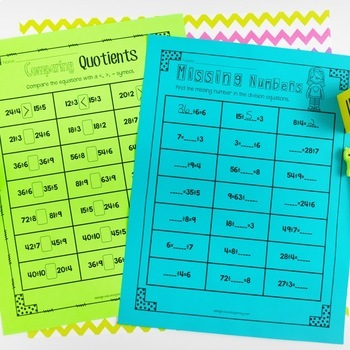No Prep Division | Printables, Activities, and Games | Print and Digital3rd - 4th, Homeschool
Subjects
Standards
Resource Type
Formats Included
• PDF
•Google Apps™
PagesThe Teacher-Author indicated this resource includes assets from Google Workspace (e.g. docs, slides, etc.).

Also included in

1. FUN Math PracticeGive your students FUN math practice with these 300+ printables! This bundle contains all six of my no prep math pacts: addition and subtraction, multiplication, division, fractions, area and perimeter, and measurement.****************************************************************
\$38.60
\$49.25
Save \$10.65

Description

Give your students FUN and rigorous division practice with these 50+ division
printables!

No Prep Needed

All you have to do is print, and you're ready to go! These division interactive worksheets are a great way to supplement your existing math curriculum. These worksheets were designed for a third grade classroom, but can be used with fourth grade students who need additional division review.

**************************************************************************************

What's Included

Division Vocabulary

Pgs. 3-4-Vocabulary Booklet

Arrays

Pg. 5-Color the Array

Pg. 6-Draw the Array

Grouping Model

Pg. 8-Draw the Grouping Model

Number Lines

Pg. 10-Complete a Number Line

Repeated Subtraction

Pg. 12-Repeated Subtraction Number Sentences

Pg. 13-Repeated Subtraction Number Sentences

Representing Division

Pg. 14-Representing Division

Pg. 15-Understanding Division

Division Facts Practice

Pg. 16-Color by Number (quotients of 2,3,4,5)

Pg. 17-Color by Number (quotients of 6,7,8,9)

Pg. 18-Color by Number (divisors of 3,4,5,6,7)

Pg. 19-Spin and Color (quotients 2-5)

Pg. 20-Spin and Color (quotients 4-7)

Pg. 21-Spin and Color (quotients 6-9)

Pg. 22-Division Graphing (quotients 2-5)

Pg. 23-Division Graphing (quotients 6-9)

Pg. 24-Division Graphing (divisors 2-5)

Pg. 25-Division Graphing (divisors 6-9)

Pgs. 26-33 (organized by factors)

Pg. 34-Find the Fact Family

Pg. 35-Division Bump (quotients of 2)

Pg. 36-Division Bump (quotients of 3)

Pg. 37-Division Bump (quotients of 4)

Pg. 38-Division Bump (quotients of 5)

Pg. 39-Division Bump (quotients of 6)

Pg. 40-Division Bump (quotients of 7)

Pg. 41-Division Bump (quotients of 8_

Pg. 42-Division Bump (quotients of 9)

Division Practice

Pg. 43-Finish the Facts (2-5)

Pg. 44-Finish the Facts (6-9)

Pg. 45-Comparing Numbers-1

Pg. 46-Comparing Numbers-2

Pg. 47-Missing Numbers

Pg. 48-Input/Output Tables

Pg. 49-Cut and Paste Fact Family

Pg. 50-Cut and Paste Repeated Subtraction

Word Problems

Pg. 51-Division Word Problems 1

Pg. 52-Division Word Problems 2

Number Bonds

Pg. 53-Dividends to 25

Pg. 54-Dividends to 81

Pgs. 55-77

Check these other math products!

No Prep Multiplication Printables

Third Grade Math Assessments Test Prep Edition

Common Core Language Arts Assessments for Third Grade

3.OA.1

3.OA.3

3.OA.4

3.OA.5

3.OA.6

3.OA.7

3.OA.9

Total Pages
Included
Teaching Duration
N/A
Report this Resource to TpT
Reported resources will be reviewed by our team. Report this resource to let us know if this resource violates TpT’s content guidelines.

Standards

to see state-specific standards (only available in the US).
Find all factor pairs for a whole number in the range 1-100. Recognize that a whole number is a multiple of each of its factors. Determine whether a given whole number in the range 1-100 is a multiple of a given one-digit number. Determine whether a given whole number in the range 1-100 is prime or composite.
Solve two-step word problems using the four operations. Represent these problems using equations with a letter standing for the unknown quantity. Assess the reasonableness of answers using mental computation and estimation strategies including rounding.
Fluently multiply and divide within 100, using strategies such as the relationship between multiplication and division (e.g., knowing that 8 × 5 = 40, one knows 40 ÷ 5 = 8) or properties of operations. By the end of Grade 3, know from memory all products of two one-digit numbers.
Apply properties of operations as strategies to multiply and divide. Examples: If 6 × 4 = 24 is known, then 4 × 6 = 24 is also known. (Commutative property of multiplication.) 3 × 5 × 2 can be found by 3 × 5 = 15, then 15 × 2 = 30, or by 5 × 2 = 10, then 3 × 10 = 30. (Associative property of multiplication.) Knowing that 8 × 5 = 40 and 8 × 2 = 16, one can find 8 × 7 as 8 × (5 + 2) = (8 × 5) + (8 × 2) = 40 + 16 = 56. (Distributive property.)
Determine the unknown whole number in a multiplication or division equation relating three whole numbers. For example, determine the unknown number that makes the equation true in each of the equations 8 × ? = 48, 5 = __ ÷ 3, 6 × 6 = ?.